• TECH INFO
• 利用接近传感器制作Arduino LED节日彩灯

TECH INFOArduino入门指南

# 利用接近传感器制作Arduino LED节日彩灯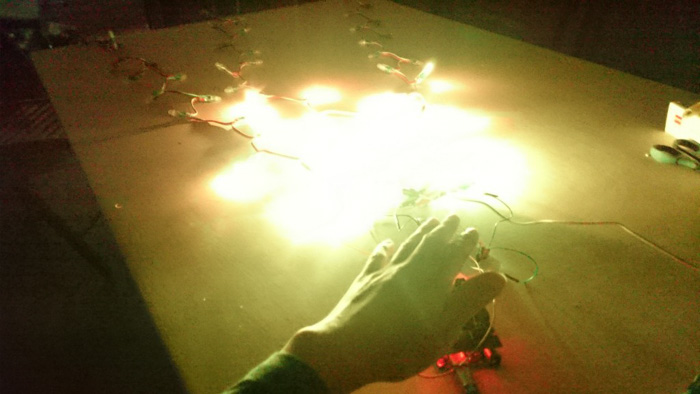1. 连接接近传感器
2. 定制程序
3. 让我们来点亮LED灯吧！

## 1.连接接近传感器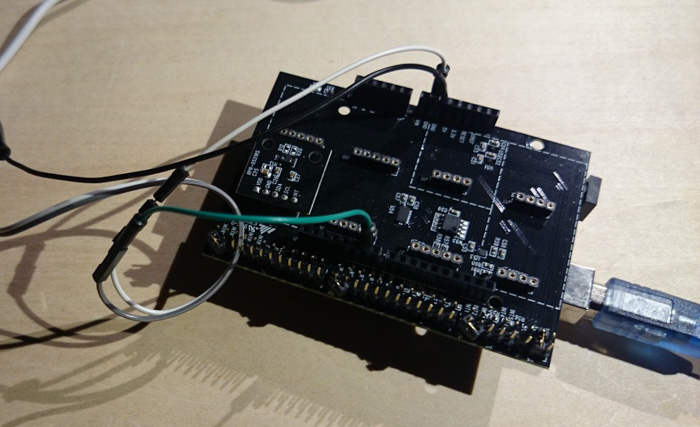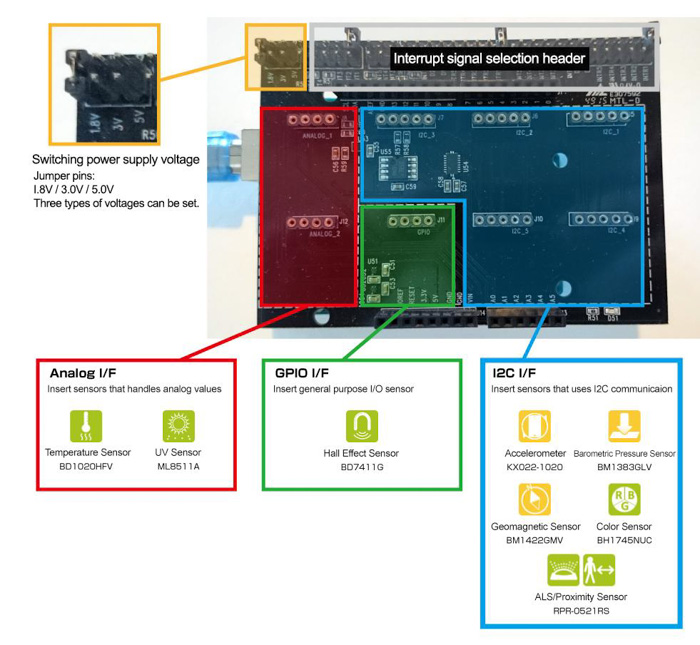LED灯串的供电需要另外的5 V电源，不能从Arduino取电。如果我们试图用Arduino的VCC或5 V为LED供电，那么Arduino可能会由于LED灯串的大电流而烧坏。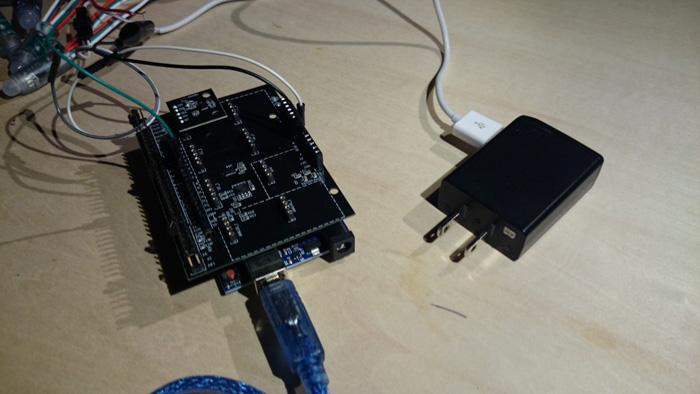LED灯串上每个LED的工作电流约为20 mA。由于供电电流为1.3A，所以可同时点亮65个LED灯。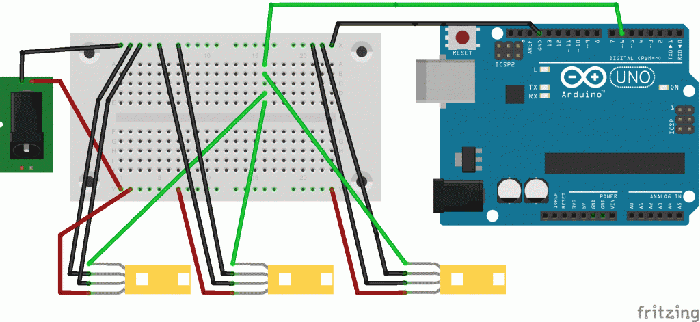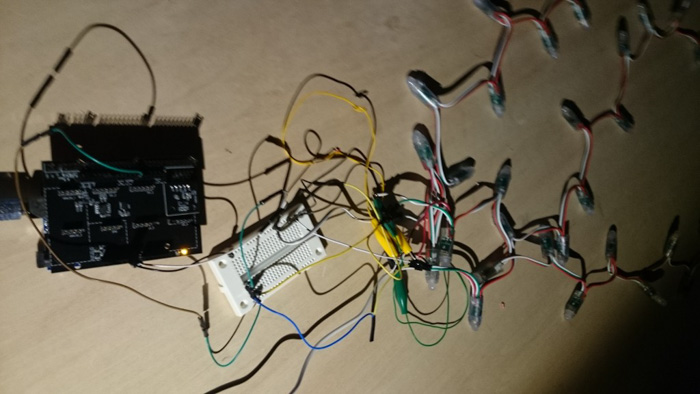## 2.定制程序

``````#include <Wire.h>

#include <RPR-0521RS.h>

#define PIN 6   //pin number of the signal terminal

#define LED_NUM 50  //number of LEDs

RPR0521RS rpr0521rs;

int wait = 200;

void setup() {

ledtape.begin();

ledtape.show();   //reflects once in all OFF state

byte rc;

Serial.begin(9600);

while (!Serial);

Wire.begin();

rc = rpr0521rs.init();

}

void loop() {

byte rc;

unsigned short ps_val;

float als_val;

byte near_far;

Serial.println("=============================");

rc = rpr0521rs.get_psalsval(&ps_val, &als_val);

//当获取传感器值时

if (rc == 0) {

Serial.print("PS:");

Serial.print(ps_val);

Serial.println();

//接近时LED灯亮起

if(ps_val > 5){

ps_val  = 500 - ps_val;

if(ps_val < 0){

ps_val = 0;

}

simpleLED(ps_val/10);

}

if (als_val != RPR0521RS_ERROR) {

Serial.print("ALS:");

Serial.print(als_val);

Serial.println();

}

}

delay(5);

}

int LEDtale;  //arrangement for the trajectory of LED

//

//逐个点亮

//

void simpleLED(int delaytime){

uint16_t i, j;

j=0;

for(i=0; i < ledtape.numPixels(); i++) {

ledtape.setPixelColor(i, rotateColor(((i) * 256 / ledtape.numPixels()) & 255));

LEDtale  = rotateColor(((i) * 256 * 9/10 / ledtape.numPixels()) & 255);

for(j=1; j < 10; j++){

LEDtale[j]  = rotateColor(((i) * 256 * (10-j)/10 / ledtape.numPixels()) & 255);

uint16_t m = i-j;

if(m < 0){

m += 50;

}

ledtape.setPixelColor(m, LEDtale[j]);

}

ledtape.show();

for(j=1; j < 10; j++){

uint16_t m = i-j;

if(m < 0){

m += 50;

}

delay(0);

ledtape.setPixelColor(m, ledtape.Color(0,0,0));

ledtape.show();

}

ledtape.setPixelColor(i, ledtape.Color(0,0,0));

ledtape.show();

}

ledtape.show();

delay(delaytime);

}

//RGB 颜色转变函数

uint32_t rotateColor(byte WheelPos) {

if(WheelPos < 85) {

return ledtape.Color(WheelPos * 3, 255 - WheelPos * 3, 0);

} else if(WheelPos < 170) {

WheelPos -= 85;

return ledtape.Color(255 - WheelPos * 3, 0, WheelPos * 3);

} else {

WheelPos -= 170;

return ledtape.Color(0, WheelPos * 3, 255 - WheelPos * 3);

}

}
``````

``````//接近时LED灯亮起

if(ps_val > 5){

ps_val  = 500 - ps_val;

simpleLED(ps_val/10); //Use the sensor value to light the LEDs

}
``````

simpleLED是点亮LED灯串的函数，但是我们现在对它进行处理，以便从LED串的开始到结束逐一点亮LED灯。这可能是不够的，所以这次我们使用一个阵列让LED灯带在一定程度上线性发光。

``int LEDtale;  //安排LED的轨迹``

## 3.让我们来点亮LED灯吧！